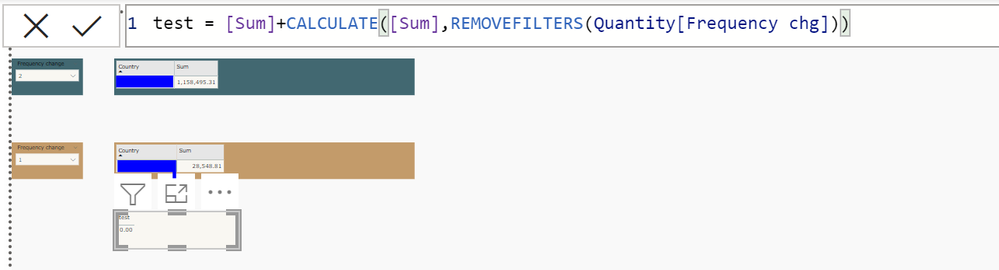cancel
Showing results for
Did you mean:Helper II

## Measure help

Sum value of measure with same slicer(repeated) and has edit interaction.

6 REPLIES 6Super User

@Aysh_2104 Sorry, having trouble following, can you post sample data as text and expected output?
Not really enough information to go on, please first check if your issue is a common issue listed here: https://community.powerbi.com/t5/Community-Blog/Before-You-Post-Read-This/ba-p/1116882

The most important parts are:
1. Sample data as text, use the table tool in the editing bar
2. Expected output from sample data
3. Explanation in words of how to get from 1. to 2.

Become an expert!: Enterprise DNA
External Tools: MSHGQM
Latest book!:
Mastering Power BI 2nd EditionDAX is easy, CALCULATE makes DAX hard...Helper II

Hello @Greg_Deckler

Here is an example :-You can see the edit interaction between the two rows, it has same slicer but works according to the edit interaction. I want the sum of these two numbers that works according to the slicer change.Super User

@Aysh_2104 Well, assuming Sum is a measure, maybe:

``Measure = [Sum] + CALCULATE([Sum],ALLEXCEPT('Table'[Country]))``

or:

``Measure = [Sum] + CALCULATE([Sum],REMOVEFILTERS('Table'[Frequency]))``

Become an expert!: Enterprise DNA
External Tools: MSHGQM
Latest book!:
Mastering Power BI 2nd EditionDAX is easy, CALCULATE makes DAX hard...Helper II

Both aren't giving me the output because of the edit interaction.Helper II

Both aren't giving me the output because of the edit interaction.Super User

@Aysh_2104 So are the slicers using the same column? If that is the case, perhaps have both slicers interact with the new visual and then do something like:

``````Measure =
VAR __Frequencies = 'Quantity'[Frequency chg]
RETURN
CALCULATE([Sum],FILTER('Quantity',[Frequency chg] IN __Frequencies))``````

Not super positive that will work and you may need to use two different tables for your slicers instead. You could a table of frequencies using using DISTINCT.

Become an expert!: Enterprise DNA
External Tools: MSHGQM
Latest book!:
Mastering Power BI 2nd EditionDAX is easy, CALCULATE makes DAX hard...